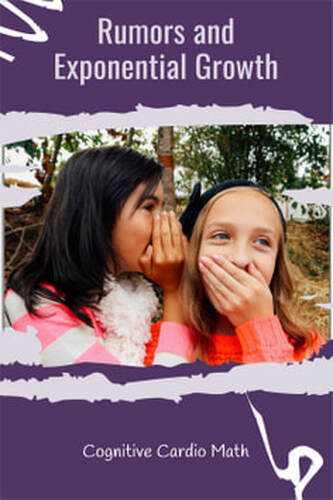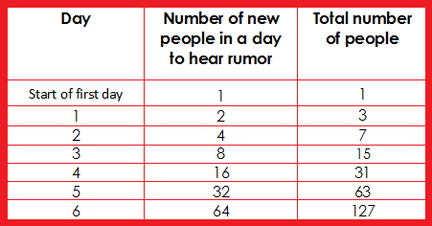# Rumors and Exponential Growth

## Discovering Exponential Growth in Middle School MathNeed an exponential growth activity for your middle school math classes?
Rumors is a great exponential growth lesson from Mathline!
This lesson allows students to explore exponential growth, in the context of spreading a rumor.

In addition to the focus on math concepts, this lesson can also help students understand how quickly rumors can actually spread….an important idea for middle schoolers to consider.

## Exponential Growth Lesson

To begin this exponential growth lesson, students are presented with the following scenario:
“Two students who were both born on December 21st, the date of the winter solstice, decide that it would be great not to have to attend school on that day. Therefore, they start a rumor that schools will be closed to celebrate the winter solstice. So, on December 1st, one of the students told two of her friends that school would be closed. On the next day, each of these students tells 2 students and on consecutive days, each of the new students tells 2 more students and so on. If there are 8,000 students in the school district, the question arises as to whether the rumor was started early enough for everyone to have heard it?”

Students can act out this scenario by having students form a human triangle, with Student A first, then the two students she told (students B and C), then four students representing the two that Student B told and the two that Student C told, etc (as far as possible, depending on how many students are in the class). This will help students visualize the problem and understand how this rumor is being spread. The triangle also help students to understand the growth pattern.

The human triangle will only go so far,  so students will then need to use their calculators or paper and pencil to find how many days it will take for the rumor to reach 8,000 people. I would recommend providing the students with a blank chart to give some structure to the students’ work after they try the human triangle.

## Exponential Growth ChartThe chart pictured here includes numbers for the first several days (the numbers for the entire chart can be found in the lesson).

In addition to understanding  more about exponential growth, students can be asked to determine the algebraic expression to describe the number of new people to hear the rumor each day, as well as the expression for the total number of people.

To read the full exponential growth lesson and the possible extensions, check out the lesson here.

## EllieWelcome to Cognitive Cardio Math! I’m Ellie, a wife, mom, grandma, and dog ‘mom,’ and I’ve spent just about my whole life in school! With nearly 30 years in education, I’ve taught:

• All subject areas in 4th and 5th grades
• Math, ELA, and science in 6th grade (middle school)

I’ve been creating resources for teachers since 2012 and have worked in the elearning industry for about five years as well!